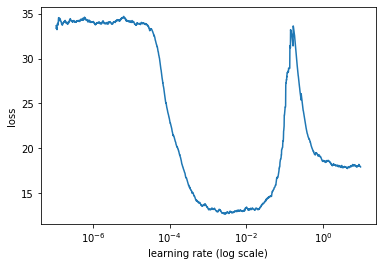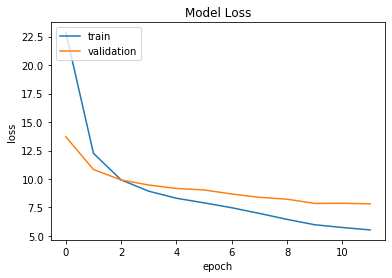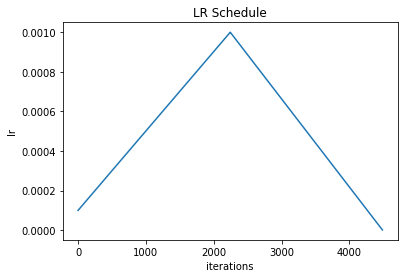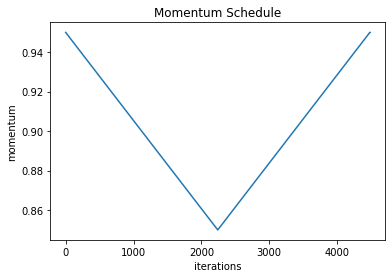In :
%reload_ext autoreload
%matplotlib inline
import os
os.environ["CUDA_DEVICE_ORDER"]="PCI_BUS_ID";
os.environ["CUDA_VISIBLE_DEVICES"]="0";


## Text Regression with Extra Regressors: An Example of Using Custom Data Formats and Models in ktrain¶

This notebook illustrates how one can construct custom data formats and models for use in ktrain. In this example, we will build a model that can predict the price of a wine by both its textual description and the winery from which it was produced. This example is inspired by FloydHub's regression template for wine price prediction. However, instead of using the wine variety as the extra regressor, we will use the winery.

Text classification (or text regression) with extra predictors arises across many scenarios. For instance, when making a prediction about the trustworthiness of a news story, one may want to consider both the text of the news aricle in addition to extra metadata such as the news publication and the authors. Here, such models can be built.

The dataset in CSV format can be obtained from Floydhub at this URL. We will begin by importing some necessary modules and reading in the dataset.

In :
# import some modules and read in the dataset
import pandas as pd
from tensorflow import keras
import numpy as np
import math
path = 'data/wine/wine_data.csv'  # ADD path/to/dataset
data = data.sample(frac=1., random_state=42)

Out:
Unnamed: 0 country description designation points price province region_1 region_2 variety winery
82956 82956 Spain Spiced apple and dried cheese aromas are simul... Mercat Brut 84 12.0 Catalonia Cava NaN Sparkling Blend El Xamfrà
60767 60767 US A little too sharp and acidic, with jammy cher... NaN 82 9.0 California California California Other Shiraz Woodbridge by Robert Mondavi
123576 123576 Spain Starts out rustic and leathery, with hints of ... Selección 12 Crianza 89 15.0 Levante Jumilla NaN Red Blend Bodegas Luzón
71003 71003 Chile Ripe to the point that it's soft and flat. Big... NaN 82 8.0 Maule Valley NaN NaN Chardonnay Melania
78168 78168 Italy From one of the best producers in the little-t... Contado Riserva 88 17.0 Southern Italy Molise NaN Aglianico Di Majo Norante

## Cleaning the Data¶

We use the exact same data-cleaning steps employed in FloydHub's regression example for this dataset.

In :
# Clean it from null values
data = data[pd.notnull(data['country'])]
data = data[pd.notnull(data['price'])]
data = data.drop(data.columns, axis=1)
variety_threshold = 500 # Anything that occurs less than this will be removed.
value_counts = data['variety'].value_counts()
to_remove = value_counts[value_counts <= variety_threshold].index
data.replace(to_remove, np.nan, inplace=True)
data = data[pd.notnull(data['variety'])]
data = data[pd.notnull(data['winery'])]

# Split data into train and test
train_size = int(len(data) * .8)
print ("Train size: %d" % train_size)
print ("Test size: %d" % (len(data) - train_size))

# Train features
description_train = data['description'][:train_size]
variety_train = data['variety'][:train_size]

# Train labels
labels_train = data['price'][:train_size]

# Test features
description_test = data['description'][train_size:]
variety_test = data['variety'][train_size:]

# Test labels
labels_test = data['price'][train_size:]

x_train = description_train.values
y_train = labels_train.values
x_test = description_test.values
y_test = labels_test.values

# winery  metadata to be used later
winery_train = data['winery'][:train_size]
winery_test = data['winery'][train_size:]

Train size: 95612
Test size: 23904


## Building a Vanilla Text Regression Model in ktrain¶

We will preprocess the data and select a linreg model for our initial "vanilla" text regression model.

In :
import ktrain
from ktrain import text

using Keras version: 2.2.4-tf

In :
trn, val, preproc = text.texts_from_array(x_train=x_train, y_train=y_train,
x_test=x_test, y_test=y_test,
ngram_range=3,
maxlen=200,
max_features=35000)

task: text regression (supply class_names argument if this is supposed to be classification task)
language: en
Word Counts: 30807
Nrows: 95612
95612 train sequences
train sequence lengths:
mean : 41
95percentile : 62
99percentile : 74
max_features changed to 1765149 with addition of ngrams
Average train sequence length with ngrams: 120
train (w/ngrams) sequence lengths:
mean : 121
95percentile : 183
99percentile : 219
x_train shape: (95612,200)
y_train shape: 95612
23904 test sequences
test sequence lengths:
mean : 41
95percentile : 62
99percentile : 74
Average test sequence length with ngrams: 111
test (w/ngrams) sequence lengths:
mean : 112
95percentile : 171
99percentile : 207
x_test shape: (23904,200)
y_test shape: 23904

In :
text.print_text_regression_models()

fasttext: a fastText-like model [http://arxiv.org/pdf/1607.01759.pdf]
linreg: linear text regression using a trainable Embedding layer
bigru: Bidirectional GRU with pretrained word vectors [https://arxiv.org/abs/1712.09405]
standard_gru: simple 2-layer GRU with randomly initialized embeddings
bert: Bidirectional Encoder Representations from Transformers (BERT) [https://arxiv.org/abs/1810.04805]
distilbert: distilled, smaller, and faster BERT from Hugging Face [https://arxiv.org/abs/1910.01108]

In :
model = text.text_regression_model('linreg', train_data=trn, preproc=preproc)

maxlen is 200
done.


## Adding an Extra Regressor to Our Model¶

Next, we will add an extra regressor to our model, thereby, creating a new, augmented model. We choose the winery as the extra regressor, which is a categorical variable. Instead of representing the winery as a typical one-hot-encoded vector, we will learn an embedding for the winery during training. The embedding module will then be concatenated with our linreg text regression model forming a new model. The new model expects two distinct inputs. The first input is an integer representing the winery. The second input is a sequence of word IDs - standard input to neural text classifiers/regressors.

In :
extra_train_data = winery_train
extra_test_data = winery_test

# encode winery as integers
from sklearn.preprocessing import LabelEncoder
encoder = LabelEncoder()
encoder.fit(data['winery'])
extra_train = encoder.transform(extra_train_data)
extra_test = encoder.transform(extra_test_data)
no_of_unique_cat = np.max(extra_train) + 1
embedding_size = min(np.ceil((no_of_unique_cat)/2), 50 )
embedding_size = int(embedding_size)
vocab =  no_of_unique_cat+1
print(embedding_size)
extra_train = np.expand_dims(extra_train, -1)
extra_test = np.expand_dims(extra_test, -1)

# winery module
extra_input = keras.layers.Input(shape=(1,))
extra_output = keras.layers.Embedding(vocab, embedding_size, input_length=1)(extra_input)
extra_output = keras.layers.Flatten()(extra_output)
extra_model = keras.Model(inputs=extra_input, outputs=extra_output)

# Combine winery module with linreg model
merged_out = keras.layers.concatenate([extra_model.output, model.output])
merged_out = keras.layers.Dropout(0.25)(merged_out)
merged_out = keras.layers.Dense(1000, activation='relu')(merged_out)
merged_out = keras.layers.Dropout(0.25)(merged_out)
merged_out = keras.layers.Dense(500, activation='relu')(merged_out)
merged_out = keras.layers.Dropout(0.5)(merged_out)
merged_out = keras.layers.Dense(1)(merged_out)
combined_model = keras.Model([extra_model.input] + [model.input], merged_out)
combined_model.compile(loss='mae',
metrics=['mae'])

50


## Wrapping our Data in an Instance of ktrain.Dataset¶

To use this custom data format of two inputs in ktrain, we will wrap it in a ktrain.dataset.Dataset instance. There are two ways to do this.

The first is to represent our datasets as tf.data.Dataset instances and then wrap each in a ktrain.dataset.TFDataset instance, which is a wrapper to a tf.data.Dataset. Use of tf.data.Dataset instances can potentially yield certain performance improvements. See this example notebook for a demonstration of using the ktrain.dataset.TFDataset class. For this example, one can make us of ktrain.dataset.TFDataset instances as follows:

import tensorflow as tf
from ktrain.dataset import TFDataset
BATCH_SIZE = 256

trn_combined = [extra_train] +  [trn] + [trn]
val_combined = [extra_test] + [val] + [val]

def features_to_tfdataset(examples):

def gen():
for idx, ex0 in enumerate(examples):
ex1 = examples[idx]
label = examples[idx]
x = (ex0, ex1)
y = label
yield ( (x, y) )

tfdataset= tf.data.Dataset.from_generator(gen,
((tf.int32, tf.int32), tf.int64),
((tf.TensorShape([None]), tf.TensorShape([None])), tf.TensorShape([])) )
return tfdataset
train_tfdataset= features_to_tfdataset(trn_combined)
val_tfdataset= features_to_tfdataset(val_combined)
train_tfdataset = train_tfdataset.shuffle(trn_combined.shape).batch(BATCH_SIZE).repeat(-1)
val_tfdataset = val_tfdataset.batch(BATCH_SIZE)

train_data = TFDataset(train_tfdataset, n=trn_combined.shape, y=trn_combined)
val_data = TFDataset(val_tfdataset, n=val_combined.shape, y=val_combined)
learner = ktrain.get_learner(combined_model, train_data=train_data, val_data=val_data)


The second approach is to wrap our datasets in a subclass of ktrain.dataset.SequenceDataset. We must be sure to override and implment the required methods (e.g., def nsamples and def get_y). The ktrain.dataset.SequenceDataset class is simply a subclass of tf.keras.utils.Sequence. See the TensorFlow documentation on the Sequence class for more information on how Sequence wrappers work.

We employ the second approach in this tutorial. Note that, in the implementation below, we have made MyCustomDataset more general such that it can wrap lists containing an arbitrary number of inputs instead of just the two needed in our example.

In :
class MyCustomDataset(ktrain.dataset.SequenceDataset):
def __init__(self, x, y, batch_size=32, shuffle=True):
# error checks
err = False
if type(x) == np.ndarray and len(x.shape) != 2: err = True
elif type(x) == list:
for d in x:
if type(d) != np.ndarray or len(d.shape) != 2:
err = True
break
else: err = True
if err:
raise ValueError('x must be a 2d numpy array or a list of 2d numpy arrays')
if type(y) != np.ndarray:
raise ValueError('y must be a numpy array')
if type(x) == np.ndarray:
x = [x]

# set variables
super().__init__(batch_size=batch_size)
self.x, self.y = x, y
self.indices = np.arange(self.x.shape)
self.n_inputs = len(x)
self.shuffle = shuffle

# required for instances of tf.keras.utils.Sequence
def __len__(self):
return math.ceil(self.x.shape / self.batch_size)

# required for instances of tf.keras.utils.Sequence
def __getitem__(self, idx):
inds = self.indices[idx * self.batch_size:(idx + 1) * self.batch_size]
batch_x = []
for i in range(self.n_inputs):
batch_x.append(self.x[i][inds])
batch_y = self.y[inds]
return tuple(batch_x), batch_y

# required for instances of ktrain.Dataset
def nsamples(self):
return self.x.shape

#required for instances of ktrain.Dataset
def get_y(self):
return self.y

def on_epoch_end(self):
if self.shuffle:  np.random.shuffle(self.indices)


Note that, you can also add a to_tfdataset method to your ktrain.dataset.SequenceDataset subclass. The to_tfdataset method is responsible for converting your dataset to a tf.Dataset and, if it exists, will be called by ktrain just prior to training. We have not done this here.

## Using the Custom Model and Data Format¶

Once we wrap our data in a ktrain.dataset.SequenceDataset instance, we can wrap the model and datasets in a Learner object and use ktrain normally.

In :
train_data = MyCustomDataset([extra_train] +  [trn], trn, shuffle=True)
val_data = MyCustomDataset([extra_test] + [val], val, shuffle=False)
learner = ktrain.get_learner(combined_model, train_data=train_data, val_data=val_data, batch_size=256)


### Estimate Learning Rate¶

We'll choose a learning rate where the loss is falling. As shown in the plot, 1e-3 seems to be a good choice in this case.

In :
learner.lr_find(show_plot=True, restore_weights_only=True)

simulating training for different learning rates... this may take a few moments...
Train for 373 steps
Epoch 1/1024
373/373 [==============================] - 9s 24ms/step - loss: 34.1117 - mae: 34.1153
Epoch 2/1024
373/373 [==============================] - 8s 20ms/step - loss: 28.8677 - mae: 28.8826
Epoch 3/1024
373/373 [==============================] - 8s 20ms/step - loss: 13.2890 - mae: 13.2908
Epoch 4/1024
373/373 [==============================] - 8s 21ms/step - loss: 20.4389 - mae: 20.4431
Epoch 5/1024
359/373 [===========================>..] - ETA: 0s - loss: 17.9780 - mae: 17.9780

done.### Train the Model¶

We will now train the model using the estimated learning rate from above for 12 epochs using the 1cycle learning rate policy.

In :
learner.fit_onecycle(1e-3, 12)

begin training using onecycle policy with max lr of 0.001...
Train for 374 steps, validate for 94 steps
Epoch 1/12
374/374 [==============================] - 9s 23ms/step - loss: 22.8788 - mae: 22.8866 - val_loss: 13.7107 - val_mae: 13.7028
Epoch 2/12
374/374 [==============================] - 9s 23ms/step - loss: 12.2521 - mae: 12.2531 - val_loss: 10.8341 - val_mae: 10.8276
Epoch 3/12
374/374 [==============================] - 9s 23ms/step - loss: 9.9158 - mae: 9.9183 - val_loss: 9.9131 - val_mae: 9.9106
Epoch 4/12
374/374 [==============================] - 8s 23ms/step - loss: 8.9252 - mae: 8.9264 - val_loss: 9.4691 - val_mae: 9.4692
Epoch 5/12
374/374 [==============================] - 8s 23ms/step - loss: 8.3064 - mae: 8.3072 - val_loss: 9.1714 - val_mae: 9.1709
Epoch 6/12
374/374 [==============================] - 8s 22ms/step - loss: 7.9027 - mae: 7.9037 - val_loss: 9.0367 - val_mae: 9.0353
Epoch 7/12
374/374 [==============================] - 9s 23ms/step - loss: 7.4723 - mae: 7.4741 - val_loss: 8.6807 - val_mae: 8.6820
Epoch 8/12
374/374 [==============================] - 9s 23ms/step - loss: 6.9741 - mae: 6.9762 - val_loss: 8.3878 - val_mae: 8.3916
Epoch 9/12
374/374 [==============================] - 8s 22ms/step - loss: 6.4518 - mae: 6.4508 - val_loss: 8.2264 - val_mae: 8.2321
Epoch 10/12
374/374 [==============================] - 8s 23ms/step - loss: 5.9795 - mae: 5.9803 - val_loss: 7.8524 - val_mae: 7.8609
Epoch 11/12
374/374 [==============================] - 8s 23ms/step - loss: 5.7376 - mae: 5.7394 - val_loss: 7.8682 - val_mae: 7.8760
Epoch 12/12
374/374 [==============================] - 8s 23ms/step - loss: 5.5266 - mae: 5.5273 - val_loss: 7.8161 - val_mae: 7.8243

Out:
<tensorflow.python.keras.callbacks.History at 0x7f84a50470f0>

Our final validation MAE is 7.82, which means our predictions are, on average, about $8 off the mark, which is not bad considering our model only looks at the textual description of the wine and the winery. ### Plot Some Training History¶ The validation loss is still decreasing, which suggests we could train further if desired. The second and third plot show the learning rate and momentum schedules employed by fit_onecycle. In : learner.plot('loss')In : learner.plot('lr')In : learner.plot('momentum')### View Top Losses¶ Let's examine the validation examples that we got the most wrong. Looks like our model has trouble with expensive wines. In : learner.view_top_losses(n=3)  ---------- id:21790 | loss:1042.46 | true:1100.0 | pred:57.54) ---------- id:13745 | loss:1014.34 | true:1400.0 | pred:385.66) ---------- id:11710 | loss:884.58 | true:980.0 | pred:95.42)  In : print(x_test)  Wet earth, rain-wet stones, damp moss, wild sage and very ripe pear make for a complex opening. Further sniffs reveal more citrus: both juice and zest of lemon. The palate still holds a lot of leesy yeast flavors but its phenolic richness is tempered by total citrus freshness. This is still tightly wound; leave it so it can come into its own. The warming resonance on the palate suggests it has a long future. Drink from 2019.  In : print(x_test)  A wine that has created its own universe. It has a unique, special softness that allies with the total purity that comes from a small, enclosed single vineyard. The fruit is almost irrelevant here, because it comes as part of a much deeper complexity. This is a great wine, at the summit of Champagne, a sublime, unforgettable experience.  In : preds = learner.predict(val_data)  In : preds  Out: array([385.65793], dtype=float32) ### Making Predictions¶ Lastly, we will use our model to make predictions on 5 randomly selected wines in the validation set. In : # 5 random predictions val_data.batch_size = 1 for i in range(5): idx = np.random.choice(len(x_test)) print("TEXT:\n%s" % (x_test[idx])) print() print("\tpredicted: %s" % (np.squeeze(learner.predict(val_data[idx])))) print("\tactual: %s" % (y_test[idx])) print('----------------------------------------')  TEXT: Relatively full-bodied and muscular as well as dry, this new effort from winemaker Steve Bird features plenty of brawny citrus and spice flavors that finish long. There's no real track record, so it's probably best to drink now. predicted: 18.009167 actual: 17.0 ---------------------------------------- TEXT: Very tart and spicy, with distinct notes of clove and orange peel. Citrus and apple flavors crop up unexpectedly, and the tannins have a hint of green tea about them. predicted: 20.4764 actual: 20.0 ---------------------------------------- TEXT: Dusty apple aromas are given lift courtesy of citrus notes. This feels good on the palate, with zesty acidity. Flavors of stone fruits, tropical fruits, apple and citrus meld together well, while the finish is pure and long. predicted: 15.768029 actual: 17.0 ---------------------------------------- TEXT: Smoky and savory on the nose, with saucy fruit sitting below a veil of firm oak. Runs a bit tart and racy in the mouth, where cherry and plum flavors are boosted by blazing natural acidity. Not a sour wine, but definitely crisp and racy. predicted: 15.798236 actual: 24.0 ---------------------------------------- TEXT: Textbook Gewurztraminer, done well, starting with scents of rose petals and lychees, and moving through pear and melon flavors into a finish that shows a hint of bitterness. Medium-weight and just slightly off-dry. predicted: 23.65241 actual: 16.0 ----------------------------------------  Let's look at our most expensive prediction. Our most expensive prediction ($404) is associated with an expensive wine priced at $800, which is good. However, we are ~$400 off. Again, our model has trouble with expensive wines. This is somewhat understandable since our model only looks at short textual descriptions and the winery - neither of which contain clear indicators of their exorbitant prices.

In :
max_pred_id = np.argmax(preds)
print("highest-priced prediction: %s" % (np.squeeze(preds[max_pred_id])))
print("actual price for this wine:%s" % (y_test[max_pred_id]))
print('TEXT:\n%s' % (x_test[max_pred_id]))

highest-priced prediction: 404.31885
actual price for this wine:800.0
TEXT:
The palate opens slowly, offering an initial citrus character, followed by wood and then, finally, wonderfully rich, but taut fruit. There is still a toast character here, with apricots and pear on top of the citrus, but it is still only just developing. In 10–15 years, it will be a magnificent wine.


## Making Predictions on Unseen Examples¶

In the example above, we made predictions for examples in the validation set. To make predictions for an arbitrary set of wine data, the steps are as follows:

1. Encode the winery using the same label encoder used above for validation data
2. Preprocess the wine description using the preprocess_test method. In this example, you will use preproc.preprocess_test.
3. Combine both into a ktrain.dataset.Dataset instance, as we did above.
In [ ]: